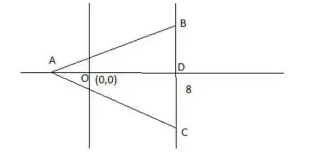# A triangle ABC has area 32 sq units | Quantitative Aptitude - Geometry - Triangles

### CAT Verbal Ability

CAT 2018 – The CAT QA section has grown increasingly difficult since 2015. In order to tackle the tougher CAT Level QA questions for the CAT Exam, it is important to understand the basics of CAT. To obtain a great CAT score, make use of MBAP Free Study material with detailed solutions and video explanations. Check out MBAP free Mock test to take these questions in a test format for free.

### You may also find remaining question solution of CAT 2018, Slot-2 by searching the question in the search bar.

The question below is from previous year CAT question from CAT 2018 exam comes from CAT Geometry– Triangles – A triangle ABC has area 32 sq units …. Find out by answering this question which tests an aspirant’s Quantitative Ability Skills:

## CAT 2018 – Slot 2 - Triangles - Question 1 - A triangle ABC has area 32 sq units

Q. 1: A triangle ABC has area 32 sq units and its side BC, of length 8 units, lies on the line x = 4. Then the shortest possible distance between A and the point (0,0) is
1. 4 units
2. 8 units
3. 4√2 units
4. 2√2 units

The distance OA will be minimum when the perpendicular from A on BC will pass through O.Area of triangle ABC = 1/2 BC×AD = 1/2×8×(AO+OD ) = 4×(AO+4)

32 = 4AO+16

AO = 4

So required minimum distance = 4Counselling Session
By IIM Mentor

### Free Material Area